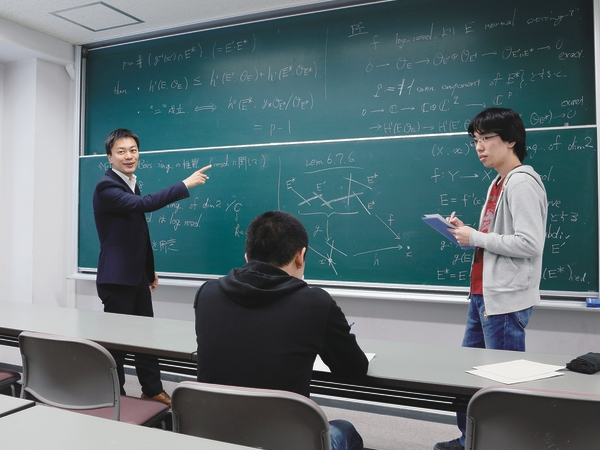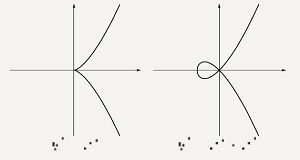Finding the meaning behind mathematical expressions - School of Science, the University of Tokyo
Oct 14, 2015

## 04. Department of Mathematics—Takagi Laboratory

Shunsuke TAKAGI
Associate Professor, Graduate School of Mathematical SciencesBS Mathematics, University of Tokyo (2000); PhD Mathematical Sciences, University of Tokyo (2004). After obtaining his PhD, Professor Takagi became a research associate at the graduate school of Kyushu University, later a project associate professor, and then an associate professor at the same institution. He has been an associate professor at the University of Tokyo since 2011 and has served as a visiting researcher at the Mathematical Sciences Research Institute (University of California, Berkeley), University of Michigan, and Massachusetts Institute of Technology.

Modern mathematics is described in highly abstract symbolic language, making it difficult for many students who struggle with mathematics to visualize what is being described. This was the case for Professor Takagi as well. He has been interested in mathematics since elementary school, but he says that when he was first exposed to some abstract concepts of mathematics in college, it was like being cast into a monochrome world. “It wasn’t until I started learning algebraic geometry that I could finally get a firm image of what those concepts were about,” he says. That was when the world of mathematics started regaining its color.

There are three major areas of mathematics: algebra deals with literal equations, geometry deals with figures, and analysis is about topics such as differentials and integrals. As its name implies, algebraic geometry is a field that involves both algebra and geometry. It combines figures with equations. More precisely, algebraic geometry is concerned with algebraic varieties, which are special types of figures that can be represented as the set of solutions of a system of polynomial equations. Takagi says that when mathematicians see a large group of things, they tend to want to divide it up into smaller classifications. When we want to classify algebraic varieties, we have to study how to handle their singularities.A singularity is a special kind of point on an algebraic variety. In Takagi’s words, they are “points that aren’t smooth, because they’re sharp or twisted.” Taking the figures to the right  as an example, the point
(x, y) = (0, 0)  is a singularity. “You can use differentials to study the properties of algebraic varieties that don’t have singularities, but this doesn’t work for ones with singularities, which therefore have been considered to be bothersome.” Several methods have been developed for working around this.

Algebraic geometry is something of a Japanese specialty. Three Japanese mathematicians have been awarded the Fields Medal, sometimes referred to as the Nobel Prize of mathematics, and all three were researchers in algebraic geometry. Two of these mathematicians studied singularities.

Heisuke Hironaka, who received Japan’s second Fields Medal in 1970, proved the existence of resolution of singularities. This means that any algebraic variety can be replaced by a similar variety that has no singularities.

Twenty years later, Japan’s third Fields Medal winner, Shigefumi Mori, completed the classification of three-dimensional algebraic varieties. Mori used a method of enumerating singularities to deal with them.

“Since the 1990s, one of the main focuses of algebraic geometry has been the classification of four-dimensional and higher algebraic varieties. But every time we go up a dimension the number of singularities increases, so enumerating each kind becomes unrealistic. What we need now is a more systematic way of dealing with singularities.” Takagi uses a theory where “adding 1 to itself a prime number of times gives 0,” a completely new approach to the study of singularities.

Professor Takagi became interested in a specific area of mathematics when he was a high school student in the early 1990s. He was first interested in topics from number theory, like Fermat’s last theorem. He says he was attracted to that theorem in particular because it was a very simple statement that had resisted all efforts to find a proof for nearly four centuries. It was finally proven in 1995, shortly before Takagi entered college. His first foray into number theory was reading Teiji Takagi’s Lectures on Elementary Number Theory.

As Gauss called number theory “the queen of mathematics,” understanding it requires all the fields of mathematics, including algebraic geometry. “Once I learned algebraic geometry, I started getting a better image of algebraic concepts. Before that, algebra had always seemed like such a dull, abstract field. Through this experience, I found algebraic geometry more fun than number theory, and here I am today.”

Mathematics is a highly individualistic field of study. Takagi shows his respect for the autonomy of his students. The only tool a mathematician needs is mathematical expressions, and mathematical expressions are a passport to the world. They provide a denser form of communication than any foreign language.

Those who have mastered the language of mathematics are able to exceed the limits of human language, and enter the world of modern mathematics.

the figure:
Examples of singularities in an algebraic variety, a polynomial plotted as a figure. In both shapes, the point (x, y) = (0, 0) is a singularity.

Student Evaluations

“He gives good explanations of things I don’t understand.” (Sho Ejiri, M1)
“He’s easy to approach, since he’s close to my age.” (Yuki Tanaka, M1)

― Office of Communication ―